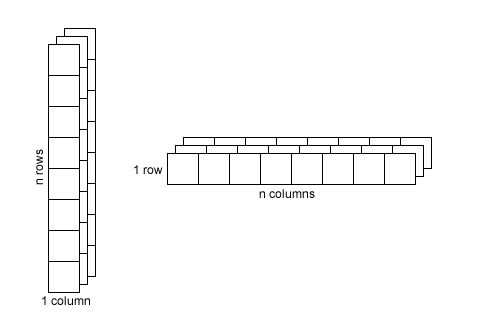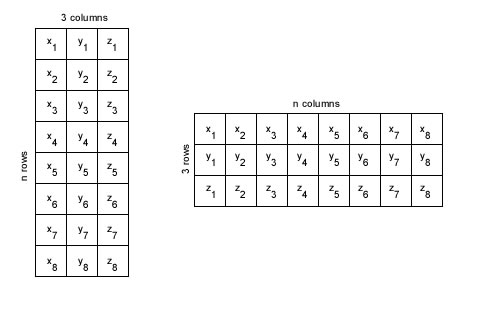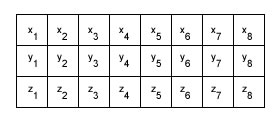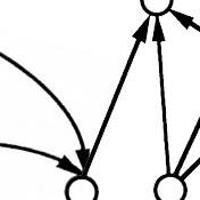## Memory layout of matrices of multi-dimensional objects

To be able to efficiently access multi-dimensional objects stored in matrices, you need to know how it's stored in the memory. How are stored in the memory depends on the format of matrix you choose. This can lead to untraceable bugs, unless you know exactly how its done and how to make way around it!

## An example

Lets take the example of a matrix storing n 3D points. There are four ways of doing this:

• n rows, 1 column, 3 channels (top left)
• 1 row, n columns, 3 channels (top right)
• n rows, 3 columns, 1 channel (bottom left)
• 3 rows, n columns, 1 channel (bottom right)

Here's a graphical way of looking at it:Now how are these stored in memory? Two key things to remember:

• Things get stored from left to right, top to bottom
• Channels are always interleaved Based on these two "rules", here's what the first one would look like:

This is the n rows, 1 column, 3 channels matrix in memory. The first triplet (x, y, z) belongs to row 1. The channels are interleaved, so you get those three values next to each other in memory. Next comes row 2, and so on. * 1 row, n columns, 3 channels would have the same layout in memory. The first triplet would be from column 1. The second from column 2, and so on. Again, channels are interleaved, so you end up getting all channels before the next column. * n rows, 3 columns, 1 channel would also have the same layout. There are no channels, so you just move from top to bottom. And you get the triplets again.

For 3 rows, n columns, 1 channel, the layout is different.In this case, you travel from left to right and top to bottom in the matrix. Since there are no channels, that's the only rule you follow. So you end up with all x's together, y's together and the z's together. Completely unlike the three other matrix formats.

## The general rule

In order to access a particular element on a matrix, here's the "formula" for the offest:

```offset = (row * numCols * numChannels) + (col * numChannels) + (channel)
```

Here, row, col and channel are the row, column and channel of the matrix you wish to access. numCols and _numChannels _are the number of columns in the matrix and number of channels in the matrix respectively.

## Working with structures

Generally, you'd want to have a matrix of structures, say, `CvPoint2D32f`. How do the above rules play out then?

Its simple, the "channels" become the `CvPoint2D32f`'s data members. So you'd have 2 "channels"... at least in the memory sort of way.

Try coming up with a layout for a matrix of matrices. ;)

## Done!

With that information, you should be able to use pointer arithmetic to move around a matrix with ease. And importantly, access the correct elements ;)

#### Related posts#### 7 unique neural network architectures